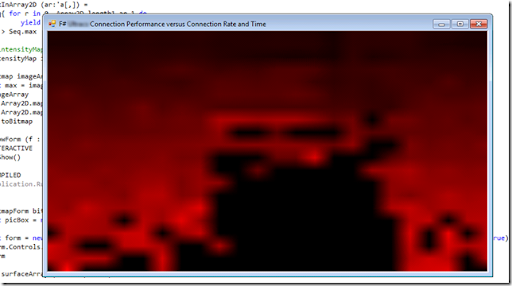## Friday, July 30, 2010

### Heat/Image Mapping with .Net/F#

As part of a demonstration to the Wellington .net user group this week I put together a demo showing a heat mapping of the performance of a transactional system as a function of time of day and arrival rate. My objective was to provide a simple eye balling test of whether performance was being impacted more by daytime customer loading or by competing overnight batch processing. I’ve tried a similar task before with Python and MatPlotLib but this time I wanted to see how easy it was to accomplish in .Net/F#.

The answer was ‘very easy’.

The interactive F# environment in VS2010 gives you JIT compiled code so it’s very fast to work with. The data structures/collections available within F# make importing and manipulating data simple – once it’s in memory you spend most of the time using filter, map and fold operations on elements – explicit recursion or iteration is rarely necessary.

I found I started with sequences then moved to arrays as data volumes increased and performance slowed. Array.Parallel.map/mapi seemed to make a significant difference whenever the operation being performed was of order a few milliseconds. It’s pointless parallising when the operation performed is short – the overhead of managing threads must just be too great.

Most of the effort was spent aggregating and binning the data, the actual image prep was trivial. The following assumes you have an array of data to display.

`open System.Drawingopen System.Drawing.Imagingopen System.Windows.Forms// adapted some code here from http://thecodedecanter.wordpress.com/2010/04/30/modelling-the-2d-heat-equation-in-f-using-100-lines-of-code/let toBitmap (arr:Color[,]) =      let image = new Bitmap(arr.GetLength(0),arr.GetLength(1),Imaging.PixelFormat.Format24bppRgb)      for i=0 to image.Width-1 do         for j=0 to image.Height-1 do             image.SetPixel(i, j, (arr.[i,j]))          done     done     image let maxInArray2D (ar:'a[,]) =    seq{ for r in 0..Array2D.length1 ar-1 do            yield Seq.max (ar.[r..r,*] |> Seq.cast<'a>)    } |> Seq.maxlet intensityMap intensity = Color.FromArgb((int (intensity * 255.0)),0,0) let bitmap imageArray =    let max = imageArray |> maxInArray2D    imageArray     |> Array2D.map (fun f -> f/max)    |> Array2D.map (fun f -> intensityMap f)    |> toBitmaplet ShowForm (f : Form) =#if INTERACTIVE    f.Show()#endif#if COMPILED    Application.Run(f)#endif let BitmapForm bitmap =     let picBox = new PictureBox(BorderStyle = BorderStyle.Fixed3D, Image = bitmap, Size = bitmap.Size,                                 Dock = DockStyle.Fill, SizeMode = PictureBoxSizeMode.StretchImage)    let form = new Form(Text = "F# Connection Performance versus Connection Rate and Time", Size = bitmap.Size, AutoSize = true)    form.Controls.Add(picBox)    formbitmap surfaceArray |> BitmapForm |> ShowForm`

If you don’t have many points that’s going to generate a small image which you can expand by dragging the corner and Windows will magically interpolate for you. I’m sure there’s a way to explicitly do this with the BitmapForm API as well – just didn’t have time to figure that out.

If you want to interpolate yourself, you could try something simple like a bilinear approach:

`let bilinearInterpolation f00 f01 f10 f11 x y =    let boundingBox = Array2D.zeroCreate<float> 2 2         boundingBox.[0,0] <- f00    boundingBox.[0,1] <- f01    boundingBox.[1,0] <- f10    boundingBox.[1,1] <- f11    let A = RowVector.ofList [1.-x; x]    let B = Matrix.ofArray2D boundingBox    let C = Vector.ofList [1.-y; y]    A * B * C`

Or why not just double the resolution?

`// take an array m x n and return (2m-1) x (2n-1)let interpolate (A:float[,]) =    let B = Array2D.zeroCreate<float> (2*(A.GetLength 0) - 1) (2*(A.GetLength 1) - 1)    for row in 0..((A.GetLength 0) - 1) do        for col in 0..((A.GetLength 1) - 1) do            B.[2*row, 2*col] <- A.[row,col]        done    done    //     for row in 0..((B.GetLength 0) - 1) do        if row % 2 = 0 then            for col in 0..((B.GetLength 1) - 1) do                if col % 2 = 1 then                    B.[row, col] <- (B.[row,col-1] + B.[row,col+1]) / 2.            done        else            for col in 0..((B.GetLength 1) - 1) do                if col % 2 = 0 then                    B.[row, col] <- (B.[row-1,col] + B.[row+1,col]) / 2.                else                    B.[row, col] <- (B.[row-1,col-1] + B.[row+1,col-1] + B.[row-1,col+1] + B.[row+1,col+1]) / 4.            done    done    B // now I can do this...bitmap (surfaceArray |> interpolate |> interpolate |> interpolate |> interpolate)|> BitmapForm |> ShowForm`

And generate results like this (where connection rate increases down, time goes across, and redness indicates duration of calls):